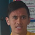## Wednesday, 30 July 2014

### 100 questions, Wednesday's

Wednesday 30th July, 2014

100 Questions:

 11 + 8 = 19 16 ÷ 8 = 2 15 ÷ 3 = 5 9  x  9 = 81 15 ÷ 5 = 3 28 ÷ 7 = 4 6 - 0 = 6 42 ÷ 6 =  7 12 + 4 = 16 12 ÷ 4 = 3 11 - 6 = 5 9 ÷ 3 = 3 6 x 5 = 30 6 + 10 = 16 7 + 6 = 13 9 - 0 = 9 0 + 1 = 1 56 ÷ 8 = 7 14 - 7 = 7 1 ÷ 1 = 1 28 ÷ 7 = 4 2 x 9 = 18 36 ÷ 9 = 4 15 ÷ 5 = 3 16 ÷ 8 = 2 1 x 5 = 5 0 x 7 = 0 10 + 11 = 21 9 - 0 = 9 9 ÷ 3 = 3 1 x 5 = 5 10 - 6 = 4 12 ÷ 6 = 2 6 - 0 = 6 25 ÷ 5 = 5 6 + 8 = 14 12 ÷ 2 = 6 7 - 3 = 4 9 - 0 = 9 9 + 12 = 21 12 ÷ 4 = 3 10 - 8 = 2 11 + 7 = 18 3 + 8 = 11 3 + 7 = 10 1 x 4 = 4 45 ÷ 5 = 9 8 x 3 = 24 32 ÷ 8 = 4 8 + 2 = 10 9 - 6 = 3 12 - 4 = 8 7 x 6 = 42 15 ÷ 5 = 3 6 x 8 = 48 10 - 8 = 2 30 ÷ 6 = 5 9 ÷ 9 = 1 4 x 4 = 16 7 - 2 = 5 13 - 8 = 5 9 - 6 = 3 8 - 3 = 5 36 ÷ 9 = 4 15 ÷ 3 = 5 5 x 3 = 15 8 x 4 = 32 4 - 2 = 2 18 ÷ 2 = 9 0 x 6 = 0 6 + 9 = 15 7 - 3 = 4 6 x 0 = 0 9 ÷ 1 = 9 10 ÷ 5 = 2 9 x 0 = 0 8 + 3 = 11 14 ÷ 7 = 2 12 ÷ 4 = 3 10 + 9 = 19 7 x 8 = 56 3 x 5 = 15 8 + 4 = 12 7 x 7 = 49 0 x 6 = 0 12 + 11 = 23 0 x 8 = 0 9 x 1 = 9 40 ÷ 5 = 8 10 - 2 = 8 12 - 4 = 8 4 x 5 = 20 10 + 9 = 19 0 x 9 = 0 11 - 3 = 8 8 - 5 = 3 8 x 2 = 16 12 - 7 = 5 1 x 4 = 4 12 ÷ 6 = 2

#### 1 comment:

1.Hi! Willy here!

That was such a smart and great subject to post about. Your 100 Questions. Nice to see someone using the web instead of their maths book, so they don't struggle with taking a picture.

Maybe next time, watch that 'Enter' button. That is a really long gap (look above).